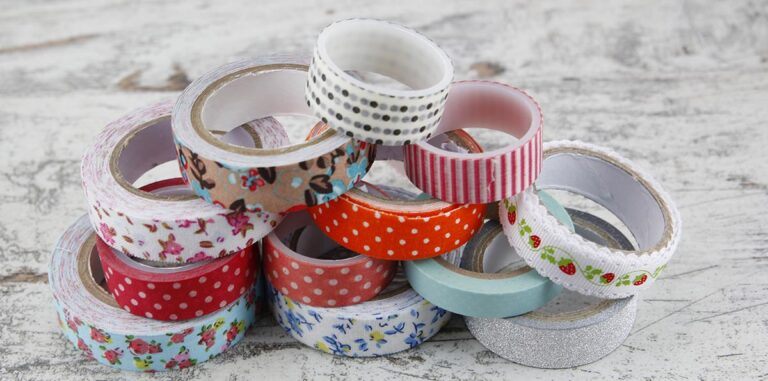# 2017 PSLE Ribbon Question & AnswerTry out this PSLE ribbon question if you are revising for your PSLE Math exam.

This Math question appeared in the PSLE 2017 Math exam paper and triggered much controversy, hitting the headlines of news sites like Mothership and Yahoo News, and was one of the most discussed question forums like KiasuParents and Reddit.

### 2017 PSLE ribbon question

Jess wants 200 ribbons of length 110 cm for a party. However, the ribbons were sold at 25 m per tape. How many tapes will Jess need?

* This might not be the exact phrasing of the question in the Primary School Leaving Examination (PSLE).

PSLE Math questions such as this is a classic example of today’s PSLE questions that are simple yet difficult to solve. Although it sounds straightforward, there are many booby traps in the question that might cause a student to get the wrong answer if they didn’t pay close attention.

### How to solve this 2017 PSLE ribbon question

To answer this PSLE ribbon question correctly, you need to take note of the units involved and apply important critical thinking skills when it comes to cutting the ribbons.

### Why is this PSLE ribbon question tricky to some students?

1. Different units of measurements
If you are too nervous during the PSLE Math exam, you might not have noticed that the different units of measurement (cm vs m).

Forgetting to convert these units of measurement will cost you some marks.

2. Misinterpreting the question
Another common mistake is to multiply 200 by 110 cm to find the total length of ribbon Jess needs and then dividing the answer by 2 500 cm to get the number of tapes needed.

This cannot be done because the leftovers of each roll of tape cannot be put together to form ribbons of 110 cm.

3. The dilemma of rounding up or down
There are 2 important points where we need to decide if we should round our answers up or down:

– When we divide 2500 cm by 110 cm to find the number of ribbons that can be cut from each tape
– After dividing the number of ribbons that we need by the number of ribbons that can be cut from one tape

Making the wrong decision at any of these points is going to cost us our answer.

### Conclusion

Did you find this PSLE 2017 Math ribbon question easy or difficult?

If you are preparing for your PSLE math paper this year, you might want to check out Practicle and learn how we can help you get better results while making math revision enjoyable with our adaptive learning game.